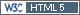## CVs for untransformed data [Software]

Hi Zhang Yong,

citing the WNL guide:
One additional parameter will appear in the Final Variance Parameters worksheet if, in addition to using the default model, the data is not transformed:
intrasubject CV = sqrt(Var(Residual)) / RefLSM

where RefLSM is the Least Squares Mean of the reference treatment.

so that's not a bugFor me even the formula above is strange. You are dividing overall standard deviation (calculated for both T and R) by LSMean of reference product only. Does that mean that if we inverse R to T, CV should change too?

Kind regards,
MittyriIng. Helmut Schütz